## November 18, 2003

### Hypothesis Testing and Confidence Intervals

We began by reviewing the stylized facts in the case.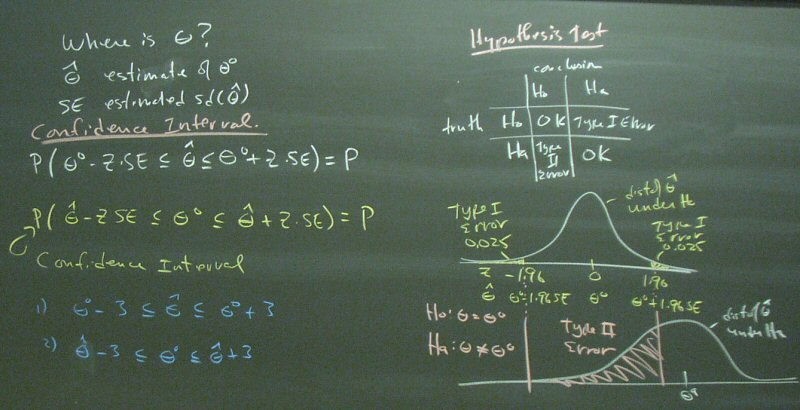Chapter 9, #22, provides a useful framework. Our discussion extended beyond the stated problem.We added a 95% confidence interval. The bottom panel shows why we use sqrt(p(1-p)/n) for the standard error of a sample proportion.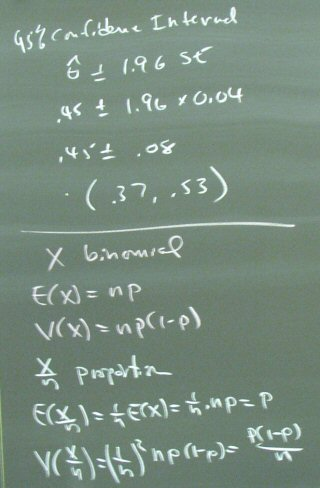Some problems give the rejection region and ask for the significance level.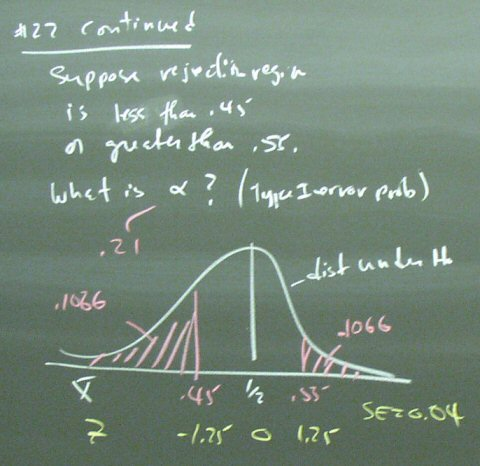Moving on, we covered the basis for a test for equality of two means.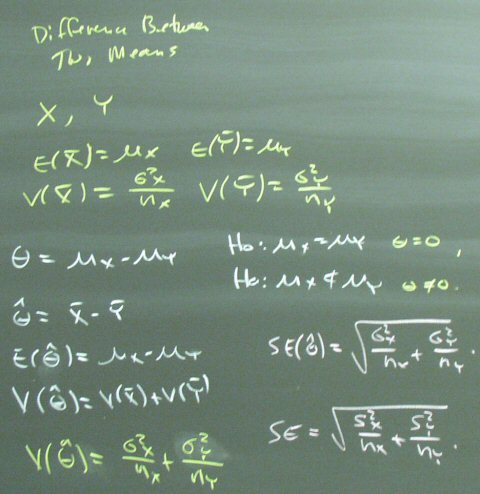Not reading the problem carefuly enough, I did Chapter 9, #26 with a 25% significance level, which was not wrong, but could just as well have been 5%. For 5%, I would have multiplied the 0.03 standard error by 1.645 to get a critical value of 0.75 - 1.645 (0.03) = 0.70.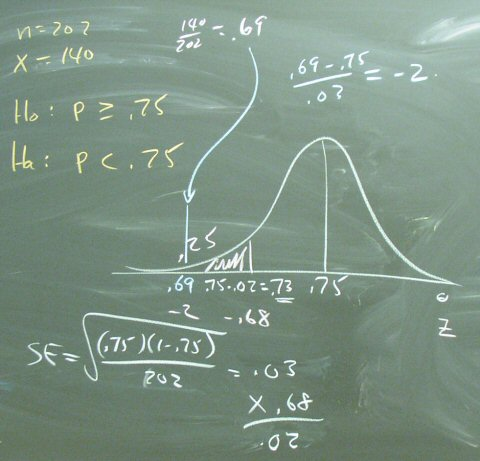Posted by bparke at 08:47 PM